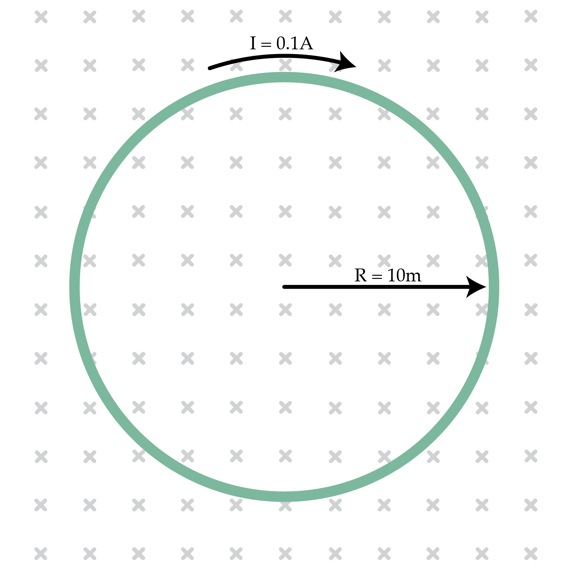# A ring in a magnetic field

A conducting ring of radius $R=10 m$ is placed in a homogeneous magnetic field, perpendicular to its plane. A constant current $I=0.1 A$ flows through the ring. It is known that the ring breaks if its tension is greater than 5N. What is the maximum magnetic field $B_{max}$ in Teslas that one can apply without breaking the ring? Neglect the magnetic field created by the current in the ring.×